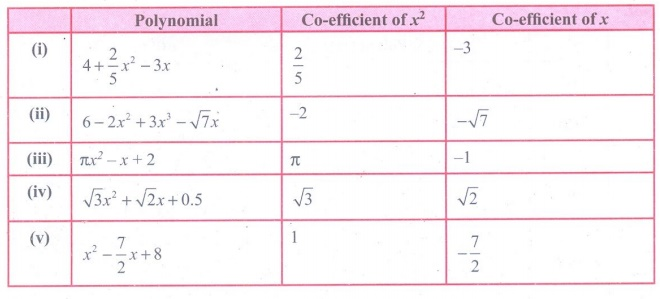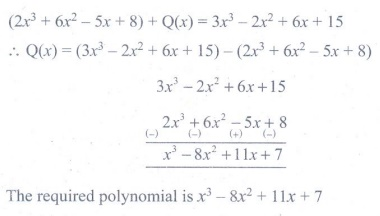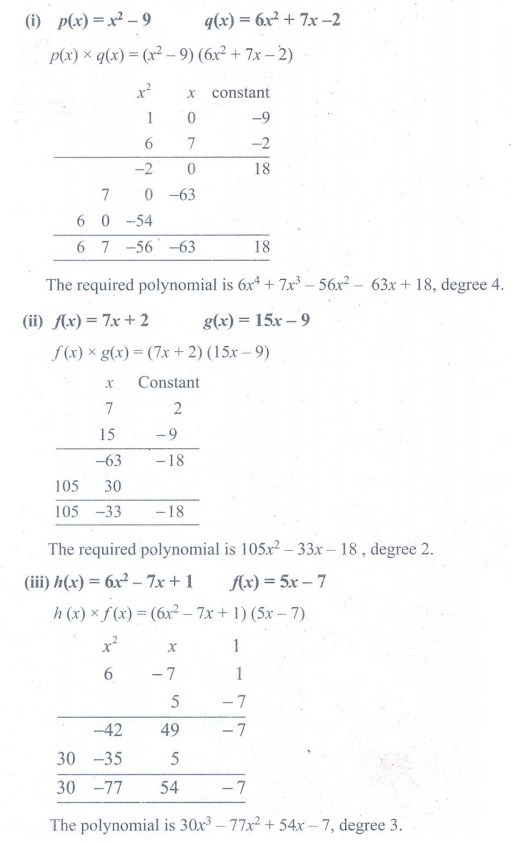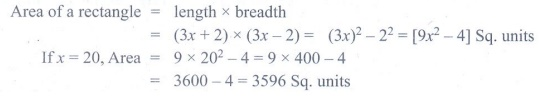Home | | Maths 9th std | Exercise 3.1: Polynomials

# Exercise 3.1: Polynomials

Maths : Algebra: Book Back, Exercise, Example Numerical Question with Answers, Solution : Exercise 3.1: Polynomials

Exercise 3.1

1. Which of the following expressions are polynomials. If not give reason:

(i) 1/x2 + 3x − 4 (ii) x2 (x − 1) (iii) 1/x (x + 5) (iv) 1/x-2 + 1/x-1 + 7 (v) √5 x2 + √3 x + √2 (vi) m23m + 7m − 102. Write the coefficient of x2 and x in each of the following polynomials.

(i) 4 + 2/5 x23x (ii) 6 − 2x2 + 3x3 − √7x (iii) πx2x + 2 (iv) √3 x2 + √2 x + 0.5 (v) x2 – 7/2 x + 83. Find the degree of the following polynomials.

(i) 1 − √2 y2 + y7 (ii) [ x3x4 + 6x6 ] / x2 (iii) x3 ( x2 + x) (iv) 3x4 + 9x2 + 27x6 (v) 2√5 p4 − 8p3/√3 + 2p2/74. Rewrite the following polynomial in standard form.

(i) x − 9 + √7x3 + 6x2 (ii) √2x2 – 7/2 x4 + x − 5x3 (iii) 7x3 – 6/5 x2 + 4x − 1 (iv) y2 + √5y311 – 7/3y + 9y45. Add the following polynomials and find the degree of the resultant polynomial.

(i) p (x) = 6x2 − 7x + 2 ; q(x) = 6x3 − 7x + 15

(ii) h (x) = 7x3 − 6x + 1 ; f(x) = 7x2 + 17x − 9

(iii) f(x) = 16x4 − 5x2 + 9 ;  g(x) = − 6x3 + 7x – 156. Subtract the second polynomial from the first polynomial and find the degree of the resultant polynomial.

(i) p(x) = 7x2 + 6x – 1 ;  q ( x) = 6x – 9

(ii) f (y) = 6y27y + 2 ; g ( y) = 7y + y3

(iii) h ( z) = z56z4 + z ; f ( z) = 6z2 + 10z – 77. What should be added to 2x3 + 6x2 − 5x + 8 to get 3x3 − 2x2 + 6x + 15 ?8. What must be subtracted from 2x4 + 4x2 − 3x + 7 to get 3x3x2 + 2x + 1 ?9. Multiply the following polynomials and find the degree of the resultant polynomial:

(i) p ( x) = x2 – 9 ; q ( x) = 6x2 + 7x – 2

(ii) f ( x) = 7x + 2 ; g (x ) = 15x – 9

(iii) h ( x) = 6x27x + 1 ; f ( x) = 5x – 710. The cost of a chocolate is Rs. (x + y) and Amir bought (x + y) chocolates. Find the total amount paid by him in terms of x and y. If x =10, y =5 find the amount paid by him.11. The length of a rectangle is (3x+2) units and it’s breadth is (3x–2) units. Find its area in terms of x. What will be the area if x = 20 units.12. p (x) is a polynomial of degree 1 and q (x)  is a polynomial of degree 2. What kind of the polynomial p(x) × q(x) is ?Tags : Numerical Problems with Answers, Solution | Algebra | Maths , 9th Maths : UNIT 3 : Algebra
Study Material, Lecturing Notes, Assignment, Reference, Wiki description explanation, brief detail
9th Maths : UNIT 3 : Algebra : Exercise 3.1: Polynomials | Numerical Problems with Answers, Solution | Algebra | Maths